# Texas Go Math Grade 4 Lesson 10.1 Answer Key Divide Using Repeated Subtraction

Refer to our Texas Go Math Grade 4 Answer Key Pdf to score good marks in the exams. Test yourself by practicing the problems from Texas Go Math Grade 4 Lesson 10.1 Answer Key Divide Using Repeated Subtraction.

## Texas Go Math Grade 4 Lesson 10.1 Answer Key Divide Using Repeated Subtraction

Essential Question

How can you use repeated subtraction and multiples to find quotients?

Investigate

Materials

• counters
• grid paper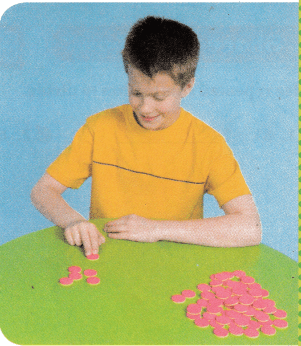John is building a backyard pizza oven with an arch opening. He has 72 bricks. He will place 6 bricks at a time as he builds the oven. If he arranges the bricks in piles of 6, how many piles will he have?

You can use repeated subtraction to divide 72 ÷ 6.

A. Begin with 72 counters. Subtract 6 counters.
How many are left? ___________

72 ÷ 6 = 12

B. Record the subtraction on grid paper as shown.
Record the number of counters left and the number of times you subtracted.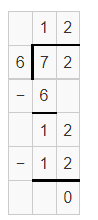C. Can you reach zero evenly? Explain.

Yes, we can reach zero evenly.

Quotient : 12

Remainder : 0

D. Count the number of times you subtracted 6 counters. ____________
So, there are ________ piles of 6 bricks.

The number of time I subtracted 6 counters = 12

So, there are 12 piles of 6 bricks.

Make Connections

Another way to divide by repeated subtraction is to use a number line. Count back by 4s from 52 to find 52 ÷ 4.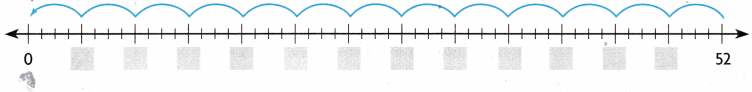How many equal groups of 4 did you subtract? _________
So, 52 ÷ 4 = _________13 equal groups of 4 is subtracted.

54÷4 = 13.5

Share and Show

Use repeated subtraction to divide.

Question 1.
84 ÷ 7 _________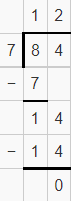Quotient = 12

Remainder = 0

Question 2.
60 ÷ 4 _________Quotient = 15

Remainder=0

Question 3.
91 ÷ 8 _________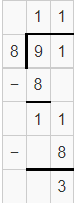Quotient = 11

Remainder = 3

Draw a number line to divide.

Question 4.
65 ÷ 5 = _________Quotient = 13

Remainder = 0

Question 5.
78 ÷ 6 = _________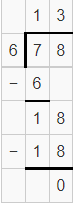Quotient = 13

Remainder= 0

Question 6.
H.O.T. Write Math Can you divide 32 by 3 evenly? Use the number line to explain your answer.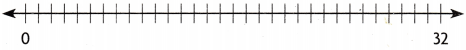Answer: Yes, we can divide 32 by 3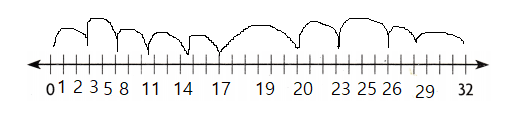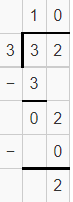Unlock the Problem

Question 7.H.O.T. Multi-Step Communicate A new playground will be 108 feet long. Builders need to allow 9 feet of space for each piece of climbing equipment. They want to put as many climbers along the length of the playground as possible. How many climbers can they place?

a. What are you asked to find?

According to the given information, we need to find the number of climbers can they place.

b. How can you use repeated subtraction to solve the problem?

Repeated subtraction method subtracts equal number of items from a group. In this problem we have to subtract 9 from 108 as many times to get the final answer.

c. How could you use multiples of the divisor to solve the problem in fewer steps?

We divide the problem with multiples of 9 to complete the problem in fewer steps.

d. Show steps to solve the problem.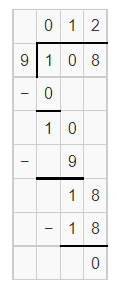Quotient : 12

Remainder : 0

Quotient : 12

Remainder: 6

e. Complete the sentences.
There are _________ equal parts of the playground, each _________ feet long. So, _________ climbers can fit along the length of the playground.

There are 108 equal parts of the playground, each 9 feet long. So, 12 climbers can fit along the length of the playground.

Question 8.
Multi-Step Analyze There are 128 students in the fourth grade and 114 students in the fifth grade. Half of these students can use the playground at the same time. How many students is that?

The number of students in the fourth grade = 128

The number of students in fifth grade = 114

Total number of students = 128+114

= 242

According to given condition, half of the students means,

242/2 = 121

l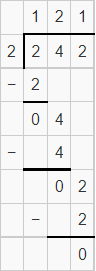Therefore, 121 students can play at the same time.

Question 9.
H.O.T. Multi-Step There are 84 seats in one section of the auditorium. Half the seats are in rows of 6 seats and the other half are in rows of 7 seats. How many rows of seats are there in that section all together?

Total number of seats in one section of the auditorium= 84

Question 10.
Donna watches her hamster and counts that he blinks 24 times in 3 minutes. If he blinks the same amount every minute, how many times does he blink in 1 minute?
(A) 6 times
(B) 8 times
(C) 7 times
(D) 5 times

Given, the number of times Donna blinks in 3 minutes = 24

Now, According to given condition,

If he blinks the same amount every minute

Now, 24/3

= 8

Therefore, He blinks 8 times in 1 minute.

Question 11.
Mrs. Morse makes gift bags for her students. She has 51 boxes of pencils to share equally among her 3 classes. How many boxes of pencils does each class receive?
(A) 10
(B) 16
(C) 17
(D) 14

Total number of boxes of pencils = 51

Number of classes she shared equally = 3

Now, 51/3Therefore, the number of pencils each class receive = 17

Question 12.
Multi-Step Marcus will work for 30 minutes on his homework exercises. He plans to spend 2 minutes on each of his 5 math exercises. He will spend the rest of his time answering 4 history questions. How much time does he plan to spend on each history question?
(A) 5 minutes
(B) 7 minutes
(C) 6 minutes
(D) 4 minutes

Given,

Total amount of time = 30 minutes.

The number of math problems = 5

The number of history questions = 4

The time he spend to solve each math exercises = 2 minutes

Total time to solve math exercise = 5 x 2 = 10 minutes

Remaining time = 30 minutes – 10 minutes

= 20 minutes.

Now, the time he spend of each history section = 20 minutes/ 4 history questions.

20/4 = 5

= 5 minutes

Therefore, Marcus spend 5 minutes on each history section.

TEXAS Test Prep

Question 13.
An architect designed the school auditorium. There are 84 seats in Section A. Each row has 6 seats. How many rows of seats are in Section A?
(A) 24
(B) 14
(C) 4
(D) 60

Total number of seats in section A = 84

The number of seats in each row = 6 seats.

Now, Total number of rows = 84/6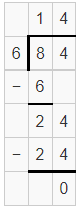### Texas Go Math Grade 4 Lesson 10.1 Homework and Practice Answer Key

Use repeated subtraction to divide.

Question 1.
65 ÷ 5 = ________Quotient = 13

Remainder = 0

Question 2.
98 ÷ 7 = ________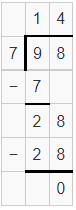Quotient = 14

Remainder = 0

Question 3.
79 ÷ 5 = ________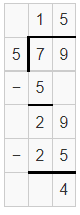Quotient = 15

Remainder = 4

Question 4.
37 ÷ 6 = ________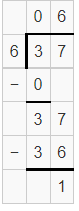Quotient = 06

Remainder = 1

Question 5.
48 ÷ 4 = ________Quotient = 12

Remainder = 0

Question 6.
59 ÷ 3 = ________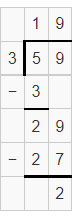quotient = 19

Remainder = 2

Draw a number line to divide.

Question 7.
45 ÷ 5 = ________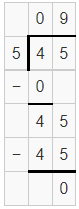Quotient = 09

Remainder = 0

Question 8.
90 ÷ 6 = ________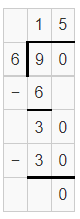Quotient = 15

Remainder = 0

Question 9.
74 ÷ 8 = ________Quotient = 09

Remainder = 2

Problem Solving

Question 10.
Last Saturday, 72 students signed up to play basketball. If there are 6 equal-sized reams, how many players are on each team?

Total number of students = 72

Number of equal sized teams = 6

Now,

72/6Therefore, there are 12 players in each team.

Question 11.
The pet store is open for 63 hours each week. If it is open 9 hours each day, how many days is it open each week?

The number of hours the pet shop open for each week = 63

The number of hours it is open each day = 9

Now, 63/9

=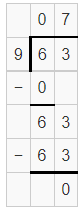Lesson Check

Fill in the bubble completely to show your answer.

Question 12.
The china shop is packing dishes to move to a new location. There are 112 blue serving bowls to pack equally into 8 boxes. How many bowls will be in each box?
(A) 13
(B) 11
(C) 12
(D) 14

The number of blue serving bowls = 112

Total number of boxes = 8

Now, 112/8 =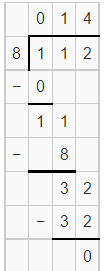Therefore, there are 14 bowls in each box.

Question 13.
There are 60 seeds in a flower package. If Carol puts an equal number of seeds in each of her 5 flowerpots, how many seeds will be in each pot?
(A) 20
(B) 12
(C) 8
(D) 15

Total number of flower packages = 60

Number of seed Carol puts in each of her flowerpots = 5

Now, 60/5 = 12Therefore, There are 12 seeds in each flower pot.

Question 14.
How many groups of 9 can you subtract from 117?
(A) 13
(B) 12
(C) 14
(D) 15

13 groups of 9 can you subtract from 117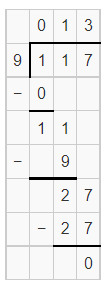Question 15.
There are 48 students in a gym class. If the students line tip in equal rows of 8, how many rows will there be?
(A) 8
(B) 6
(C) 5
(D) 7

Total number of students in gym class = 48

Number of students in each rows = 8

Now, 48/8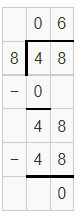Therefore, total number of rows =6

Question 16.
Multi-Step Mrs. Jenkins opened 2 boxes of crayons. Each box contains 64 crayons. She wants to divide the crayons evenly between 8 groups. How many crayons will each group receive?
(A) 18
(B) 8
(C) 16
(D) 24

Number of crayon boxes = 2

Total number of crayons in each box = 64

So, Total crayons in 2 boxes = 64+64

= 128

Given, Jenkins divide the crayons evenly between 8 groups.

Now,Therefore, each group receive 16 crayons.

Question 17.
Multi-Step John has $40 to spend at the yard sale. He buys 6 books for$2 each. He would like to spend the rest of his money on model cars for his collection. If the cars cost $7 each, how many can he buy? (A) 7 (B) 6 (C) 5 (D) 4 Answer: Total amount John have =$40

The cost of each book – $2 Total number of books = 6 So, 6 x$2 = $12 Remaining money =$40-$12$28

The cost of each car = $7 Now,$28/7

=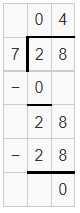Therefore, he can buy 4 cars.

Scroll to Top